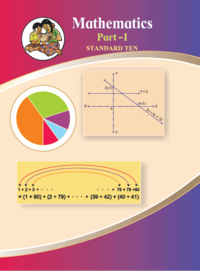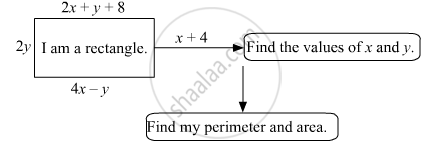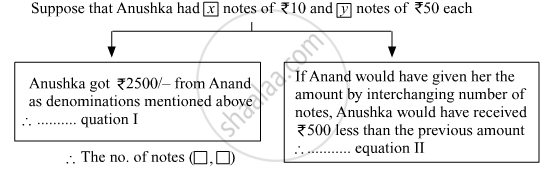SSC (English Medium) Class 10th Board ExamMaharashtra State Board
Share

# Balbharati solutions for Class 10th Board Exam Algebra chapter 1 - Linear Equations in Two Variables

## Balbharati Class 10 Mathematics 1 Algebra

#### Balbharati Balbharati Class 10 Mathematics 1 Algebra## Chapter 1: Linear Equations in Two Variables

#### Chapter 1: Linear Equations in Two Variables solutions [Pages 4 - 5]

Q 1 | Page 4

Complete the following activity to solve the simultaneous equations.
5x + 3y = 9 -----(I)
2x + 3y = 12 ----- (II)

Q 2.1 | Page 5

Solve the following simultaneous equation.
3a + 5b = 26; a + 5b = 22

Q 2.2 | Page 5

Solve the following simultaneous equation.
x + 7y = 10; 3x – 2y = 7

Q 2.3 | Page 5

Solve the following simultaneous equation.
2x – 3y = 9; 2x + y = 13

Q 2.4 | Page 5

Solve the following simultaneous equation.
5m – 3n = 19; m – 6n = –7

Q 2.5 | Page 5

Solve the following simultaneous equation.
5x + 2y = –3; x + 5y = 4

Q 2.6 | Page 5

Solve the following simultaneous equation.
$\frac{1}{3}x + y = \frac{10}{3}; 2x + \frac{1}{4}y = \frac{11}{4}$

Q 2.7 | Page 5

Solve the following simultaneous equation.
99x + 101y = 499; 101x + 99y = 501
$200x + 200y = 1000$
$\Rightarrow x + y = 5 . . . . . (III)$

Q 2.8 | Page 5

Solve the following simultaneous equation.
49x – 57y = 172; 57x – 49y = 252

#### Chapter 1: Linear Equations in Two Variables solutions [Page 8]

Q 1 | Page 8

Complete the following table to draw graph of the equations–
(I) x + y = 3 (II) x – y = 4

x + y = 3

 x 3 0 0 y 0 5 3 (x,y) (3,0) (0,5) (0,3)

x – y = 4

 x 0 -1 0 y 0 0 -4 (x,y) (0,0) (0,5) (0,-4)
Q 2.1 | Page 8

Solve the following simultaneous equations graphically.
= 6 ; – = 4

Q 2.2 | Page 8

Solve the following simultaneous equations graphically.
x + y = 5 ; x – y = 3

Q 2.3 | Page 8

Solve the following simultaneous equations graphically.
x + y = 0 ; 2x – y = 9

Q 2.4 | Page 8

Solve the following simultaneous equations graphically.
3x – y = 2 ; 2x – y = 3

Q 2.5 | Page 8

Solve the Following Simultaneous Equations Graphically.3x – 4y = –7 ; 5x – 2y = 0

Q 2.6 | Page 8

Solve the following simultaneous equations graphically.

2x – 3y = 4 ; 3y – x = 4

#### Chapter 1: Linear Equations in Two Variables solutions [Page 16]

Q 1 | Page 16

Fill in the blanks with correct number$\begin{vmatrix}3 & 2 \\ 4 & 5\end{vmatrix}$ = 3 x ____ - ____ x 4 = ____ - 8 = ____

Q 2.1 | Page 16

Find the values of following determinant.

$\begin{vmatrix}- 1 & 7 \\ 2 & 4\end{vmatrix}$
Q 2.2 | Page 16

Find the values of following determinant.

$\begin{vmatrix}5 & 3 \\ - 7 & 0\end{vmatrix}$
Q 2.3 | Page 16

Find the values of following determinant.

$\begin{vmatrix}\frac{7}{3} & \frac{5}{3} \\ \frac{3}{2} & \frac{1}{2}\end{vmatrix}$
Q 3.1 | Page 16

Solve the following simultaneous equations using Cramer’s rule.
3x – 4y = 10 ; 4x + 3y = 5

Q 3.2 | Page 16

Solve the following simultaneous equations using Cramer’s rule.
4x + 3y – 4 = 0 ; 6x = 8 – 5y

Q 3.3 | Page 16

Solve the following simultaneous equations using Cramer’s rule.
x + 2y = –1 ; 2x – 3y = 12

Q 3.4 | Page 16

Solve the following simultaneous equations using Cramer’s rule.
6x – 4y = –12 ; 8x – 3y = –2

Q 3.5 | Page 16

Solve the following simultaneous equations using Cramer’s rule.
4m + 6n = 54 ; 3m + 2n = 28

Q 3.6 | Page 16

Solve the following simultaneous equations using Cramer’s rule.
$2x + 3y = 2 ; x - \frac{y}{2} = \frac{1}{2}$

#### Chapter 1: Linear Equations in Two Variables solutions [Page 19]

Q 1.1 | Page 19

Solve the following simultaneous equations.
$\frac{2}{x} - \frac{3}{y} = 15; \frac{8}{x} + \frac{5}{y} = 77$

Q 1.2 | Page 19

Solve the following simultaneous equations.
$\frac{10}{x + y} + \frac{2}{x - y} = 4; \frac{15}{x + y} - \frac{5}{x - y} = - 2$

Q 1.3 | Page 19

Solve the following simultaneous equations.
$\frac{27}{x - 2} + \frac{31}{y + 3} = 85; \frac{31}{x - 2} + \frac{27}{y + 3} = 89$

Q 1.4 | Page 19

Solve the following simultaneous equations.
$\frac{1}{3x + y} + \frac{2}{3x - y} = \frac{3}{4}; \frac{1}{2\left( 3x + y \right)} - \frac{1}{2\left( 3x - y \right)} = - \frac{1}{8}$

#### Chapter 1: Linear Equations in Two Variables solutions [Page 26]

Q 1 | Page 26

Two numbers differ by 3. The sum of twice the smaller number and thrice the greater number is 19. Find the numbers.

Q 2 | Page 26

Complete the following.Q 3 | Page 26

The sum of father’s age and twice the age of his son is 70. If we double the age of the father and add it to the age of his son the sum is 95. Find their present ages.

Q 4 | Page 26

The denominator of a fraction is 4 more than twice its numerator. Denominator becomes 12 times the numerator, if both the numerator and the denominator are reduced by 6. Find the fraction.

Q 5 | Page 26

Two types of boxes A, B are to be placed in a truck having capacity of 10 tons. When 150 boxes of type A and 100 boxes of type B are loaded in the truck, it weighes 10 tons. But when 260 boxes of type A are loaded in the truck, it can still accommodate 40 boxes of type B, so that it is fully loaded. Find the weight of each type of box.

Q 6 | Page 26

Out of 1900 km, Vishal travelled some distance by bus and some by aeroplane. Bus travels with average speed 60 km/hr and the average speed of aeroplane is 700 km/hr. It takes 5 hours to complete the journey. Find the distance, Vishal travelled by bus.

#### Chapter 1: Linear Equations in Two Variables solutions [Pages 27 - 28]

Q 1.1 | Page 27

Choose correct alternative for the following question.
To draw graph of 4x +5y = 19, Find y when x = 1.

 A)  4 (B)  3 (C)  2 (D)  –3
Q 1.2 | Page 27

Choose correct alternative for the following question.
For simultaneous equations in variables x and y, D= 49, Dy = –63, D = 7 then what is x ?

 (A) (B) (C) (D) 7 -7 $\frac{1}{7}$ $\frac{- 1}{7}$
Q 1.3 | Page 27

Choose correct alternative for the following question.
Find the value of $\begin{vmatrix}5 & 3 \\ - 7 & - 4\end{vmatrix}$

 A)  –1 (B)  –41 (C)  41 (D)  1

Q 1.4 | Page 27

Choose correct alternative for the following question.
To solve x + y = 3 ; 3x – 2y – 4 = 0 by determinant method find D.

 A)  5 (B)  1 (C)  –5 (D)  –1
Q 1.5 | Page 27

Choose correct alternative for the following question.
ax + by = c and mx + ny = d and an ≠ bm then these simultaneous equations have -

 (A) Only one common solution. (A) No solution. (C) Infinite number of solutions. (D) Only two solution.
Q 2 | Page 27

Complete the following table to draw the graph of 2x –  6y = 3

 x -5 x y x 0 (x,y) (-5,x) (x,0)
Q 3.1 | Page 27

Solve the following simultaneous equation graphically.
2x + 3y = 12 ; x – y = 1

Q 3.2 | Page 27

Solve the following simultaneous equation graphically.
x – 3y = 1 ; 3x – 2y + 4 = 0

Q 3.3 | Page 27

Solve the following simultaneous equation graphically.
5x – 6y + 30 = 0 ; 5x + 4y – 20 = 0

Q 3.4 | Page 27

Solve the following simultaneous equation graphically.
3x – y – 2 = 0 ; 2x + y = 8

Q 3.5 | Page 27

Solve the following simultaneous equation graphically.
3x + y = 10 ;  x – y = 2

Q 4.1 | Page 27

Find the value of the following determinant.

$\begin{vmatrix}4 & 3 \\ 2 & 7\end{vmatrix}$
Q 4.2 | Page 27

Find the value of the following determinant.

$\begin{vmatrix}5 & - 2 \\ - 3 & 1\end{vmatrix}$
Q 4.3 | Page 27

Find the value of the following determinant.

$\begin{vmatrix}3 & - 1 \\ 1 & 4\end{vmatrix}$
Q 5.1 | Page 28

Solve the following equations by Cramer’s method.
6x – 3y = –10 ; 3x + 5y – 8 = 0

Q 5.2 | Page 28

Solve the following equation by Cramer’s method.
4m – 2n = –4 ; 4m + 3n = 16

Q 5.3 | Page 28

Solve the following equations by Cramer’s method.
3x – 2= $\frac{5}{2}$ ; $\frac{1}{3}x + 3y = - \frac{4}{3}$

Q 5.4 | Page 28

Solve the following equations by Cramer’s method.
7x + 3y = 15 ; 12y – 5x = 39

Q 5.5 | Page 28

Solve the following equations by Cramer’s method.
$\frac{x + y - 8}{2} = \frac{x + 2y - 14}{3} = \frac{3x - y}{4}$

Q 6.1 | Page 28

Solve the following simultaneous equations.
$\frac{2}{x} + \frac{2}{3y} = \frac{1}{6} ; \frac{3}{x} + \frac{2}{y} = 0$

Q 6.2 | Page 28

Solve the following simultaneous equations.
$\frac{7}{2x + 1} + \frac{13}{y + 2} = 27 ; \frac{13}{2x + 1} + \frac{7}{y + 2} = 33$

Q 6.3 | Page 28

Solve the following simultaneous equations.

$\frac{148}{x} + \frac{231}{y} = \frac{527}{xy} ; \frac{231}{x} + \frac{148}{y} = \frac{610}{xy}$
Q 6.4 | Page 28

Solve the following simultaneous equations.

$\frac{7x - 2y}{xy} = 5 ; \frac{8x + 7y}{xy} = 15$
Q 6.5 | Page 28

Solve the following simultaneous equations.

$\frac{1}{2\left( 3x + 4y \right)} + \frac{1}{5\left( 2x - 3y \right)} = \frac{1}{4} ; \frac{5}{\left( 3x + 4y \right)} - \frac{2}{\left( 2x - 3y \right)} = - \frac{3}{2}$
Q 7.1 | Page 28

Solve the following word problem.
A two digit number and the number with digits interchanged add up to 143. In the given number the digit in unit’s place is 3 more than the digit in the ten’s place. Find the original number.

Q 7.2 | Page 28

Solve the following word problem.
Kantabai bought $1\frac{1}{2}$ kg tea and 5 kg sugar from a shop. She paid Rs 50 as return fare for rickshaw. Total expense was Rs 700. Then she realised that by ordering online the goods can be bought with free home delivery at the same price. So next month she placed the order online for 2 kg tea and 7 kg sugar. She paid Rs 880 for that. Find the rate of sugar and tea per kg.

Q 7.3 | Page 28

Solve the following word problem.
To find number of notes that Anushka had, complete the following activity.Q 7.4 | Page 28

Solve the Following Word Problem.
Sum of the present ages of Manish and Savita is 31. Manish’s age 3 years ago was 4 times the age of Savita. Find their present ages.

Q 7.5 | Page 28

Solve the Following Word Problem.
In a factory the ratio of salary of skilled and unskilled workers is 5 : 3. Total salary of one day of both of them is Rs 720. Find daily wages of skilled and unskilled workers.

Q 7.6 | Page 28

Solve the Following Word Problem.
Places A and B are 30 km apart and they are on a st raight road. Hamid travels from A to B on bike. At the same time Joseph starts from B on bike, travels towards A. They meet each other after 20 minutes. If Joseph would have started from B at the same time but in the opposite direction (instead of towards A) Hamid would have caught him after 3 hours. Find the speed of Hamid and Joseph.

## Chapter 1: Linear Equations in Two Variables

#### Balbharati Balbharati Class 10 Mathematics 1 Algebra## Balbharati solutions for Class 10th Board Exam Algebra chapter 1 - Linear Equations in Two Variables

Balbharati solutions for Class 10th Board Exam chapter 1 (Linear Equations in Two Variables) include all questions with solution and detail explanation. This will clear students doubts about any question and improve application skills while preparing for board exams. The detailed, step-by-step solutions will help you understand the concepts better and clear your confusions, if any. Shaalaa.com has the Maharashtra State Board Balbharati Class 10 Mathematics 1 Algebra solutions in a manner that help students grasp basic concepts better and faster.

Further, we at Shaalaa.com provide such solutions so that students can prepare for written exams. Balbharati textbook solutions can be a core help for self-study and acts as a perfect self-help guidance for students.

Concepts covered in Class 10th Board Exam Algebra chapter 1 Linear Equations in Two Variables are Linear Equations in Two Variables Applications, Cramer'S Rule, Cross - Multiplication Method, Substitution Method, Elimination Method, Equations Reducible to a Pair of Linear Equations in Two Variables, Simple Situational Problems, Inconsistency of Pair of Linear Equations, Consistency of Pair of Linear Equations, Introduction of System of Linear Equations in Two Variables, Graphical Method of Solution of a Pair of Linear Equations, Determinant of Order Two.

Using Balbharati Class 10th Board Exam solutions Linear Equations in Two Variables exercise by students are an easy way to prepare for the exams, as they involve solutions arranged chapter-wise also page wise. The questions involved in Balbharati Solutions are important questions that can be asked in the final exam. Maximum students of Maharashtra State Board Class 10th Board Exam prefer Balbharati Textbook Solutions to score more in exam.

Get the free view of chapter 1 Linear Equations in Two Variables Class 10th Board Exam extra questions for and can use Shaalaa.com to keep it handy for your exam preparation

S